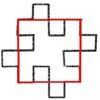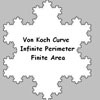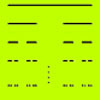#### You may also like### Squareflake

A finite area inside and infinite skin! You can paint the interior of this fractal with a small tin of paint but you could never get enough paint to paint the edge.### Von Koch Curve

Make a poster using equilateral triangles with sides 27, 9, 3 and 1 units assembled as stage 3 of the Von Koch fractal. Investigate areas & lengths when you repeat a process infinitely often.### How Many Elements Are There in the Cantor Set?

This article gives a proof of the uncountability of the Cantor set.

# Sierpinski Triangle

##### Age 16 to 18 Challenge Level:

If you break a line of length $1$ into self similar bits of length ${1\over m}$ there are $m^1$ bits and we say the dimension of the line is $1$.

If you break up a square of side $1$ into self similar squares with edge ${1\over m}$ then there are $m^2$ smaller squares and we say the dimension is $2$.

If you break up a cube of side$1$ into self similar cubes with edge ${1\over m}$ then there are $m^3$ smaller cubes and we say the dimension is $3$.

In each case we say the magnification factor is $m$ meaning that we have to scale the lengths by a factor of $m$ to produce the original shape. So the formula is: $$\text{number of bits} = \text{(magnification factor})^d$$ where $d$ is the dimension, i.e. $n = m^d$.